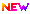High Impact Factor : 4.396| Submit Manuscript Online|

## Minimizing the Queue Overflow Probability Using Wireless Scheduling Algorithm

### Author(s):

K Anand , K M M Institute of PG studies,Tirupathi; Mr S Muni Kumar, KMM institute of PG Studies, Tirupati

### Keywords:

Wireless Scheduling Algorithm, Asymptotically Optimal Algorithms, Cellular System, Large Deviations, Queue-Over├»┬¼ŌĆÜow Probability

### Abstract

In this paper, we are interested in wireless scheduling algorithms for the downlink of a single cell that can minimize the queue-over├»┬¼ŌĆÜow probability. Speci├»┬¼┬ücally, in a large-deviation set- ting, we are interested in algorithms that maximize the asymptotic decay rate of the queue-over├»┬¼ŌĆÜow probability, as the queue-over-├»┬¼ŌĆÜow threshold approaches in├»┬¼┬ünity. We ├»┬¼┬ürst derive an upper bound on the decay rate of the queue-over├»┬¼ŌĆÜow probability over all scheduling policies. We then focus on a class of scheduling algorithms collectively referred to as the ├óŌé¼┼ōalgorithms.├óŌé¼┬Ø For a given, the -algorithm picks the user for service at each time that has the largest product of the transmission rate multiplied by the backlog raised to the power . We show that when the over├»┬¼ŌĆÜow metric is appropriately modified, the minimum-cost-to-over├»┬¼ŌĆÜow under the -algorithm can be achieved by a simple linear path, and it can be written as the solution of a vector-optimization problem. Using this structural property, we then show that when approaches in├»┬¼┬ünity, the -algorithms asymptotically achieve the largest decay rate of the queue-over├»┬¼ŌĆÜow probability. Finally, this result enables us to design scheduling algorithms that are both close to optimal in terms of the asymptotic decay rate of the over├»┬¼ŌĆÜow probability and empirically shown to maintain small queue-over├»┬¼ŌĆÜow probabilities over queue-length ranges of practical interest.

### Other Details

Paper ID: IJSRDV7I20128
Published in: Volume : 7, Issue : 2
Publication Date: 01/05/2019
Page(s): 23-26